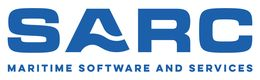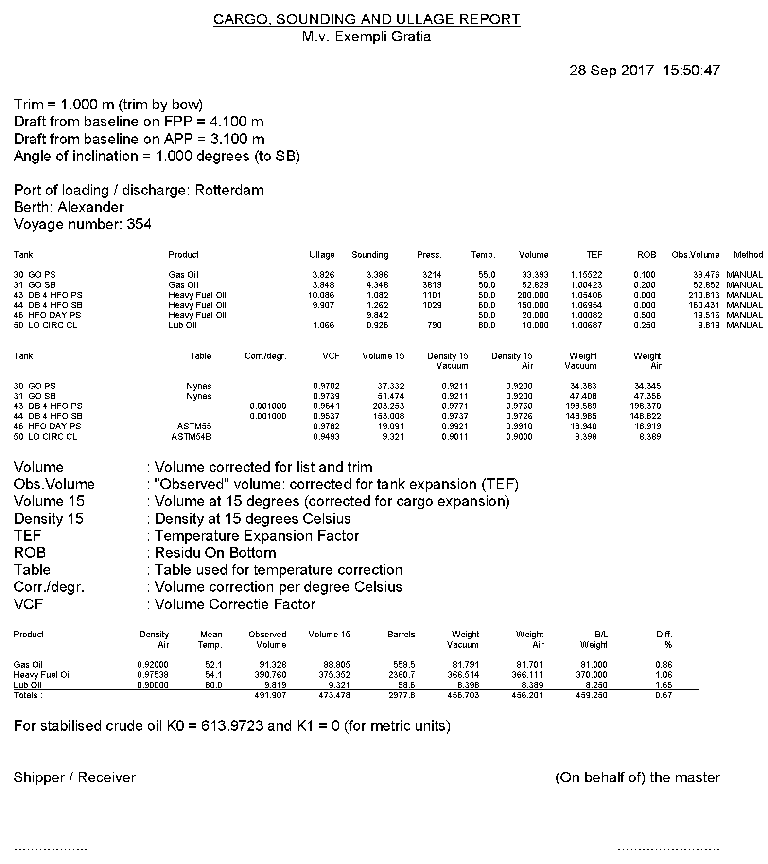PIAS Manual  2021 Program for the Integral Approach of Shipdesign
Sounding: calculate tank particulars including effects of heel and trim
This module computes, for compartments defined with Layout, tank capacities and corresponding COG for an arbitrary list/trim combination, if required also with temperature correction to account for expansion of cargo or tank structure. These results can be utilized in a cargo/ullage report or be exported to a loading condition.

# Specify list and trim

An input window pops up, where the following data can be given:

• Trim in meters (Trim by bow positive), which is the difference in draft on the FPP and the APP (Tfpp - Tapp).
• Angle of inclination in degrees only positive angles can be filled in here.
• The above given heeling angle is to PS or SB.
• Mean draft. This draft is read out by the draft sensor, which is an option in the context of LOCOPIAS. For calculating the tank capacities only, it is not required to give a draft.

# Calculate tank particulars

An input screen appears for defining all tank particulars, such as sounding or ullage, volume, density and weight. If one of these items is changed, the other items will be adjusted automatically. The ullage can only be used if a sounding pipe has been defined for the specific compartment (for which reference is made to Sounding pipe). In the alternative window the LCG, VCG, TCG and FSM can be read. By pressing <Enter>, with the cursor on the line of a compartment, a number of particulars can be defined for the specific compartment:

Tank name
As defined in the LOCOPIAS vessel model.
Include this tank in ullage report
If this compartment should be included in the cargo/ullage report (for an example see Print Cargo/Ullage report on screen) then this field should be set to ‘yes’.
Product (substance)
The name of the product, which will be used in the cargo/ullage report.
Conversion table
For the calculation of the cargo weight of heated hydrocarbons, the following conversion tables are available:
• No temperature correction.
• Correction factor per degree. The ‘Volume Correction Factor’ is calculated according to the defined temperature and the correction factor per degree (coefficient of expansion).
• Volume Correction Factor. The ‘Volume Correction Factor’ can be defined directly.
• Table 54B. The ‘Volume Correction Factor’ is determined according to ASTM table 54B.
• Table 55. The ‘Volume Correction Factor’ is determined according to ASTM table 55.
This is the value that is sent by the tank measurement system ( Import tank data from tank measurement systeem). The data link value is for checking purposes only.
Temperature
The standard temperature is 15° Celsius. The volume is determined at this temperature. The actual temperature of the substance can be defined here.
Volume (not corrected for expansion)
This is the volume that is calculated according to the sounding or ullage for this compartment. This volume comes from the previous window with the list of all the compartments.
Density at 15° Celsius (in air)/(in vacuum)
The density of the substance at 15° Celsius can be defined here. If the density in air is defined, the density in vacuum is calculated automatically. These two densities are connected to each other and cannot be defined separately.
Correction factor per degree Celsius
This factor is used if the conversion table ‘Correction factor per degree’ has been selected, and calculates the volume correction factor.
Volume Correction Factor
This factor can be determined with four different methods:
• This factor is defined manually, using conversion table ‘Volume Correction Factor’.
• This factor is calculated with the correction factor per degree and the difference between the standard and actual temperature. The conversion table ‘Correction factor per degree’ must be selected.
• This factor is read out from the conversion table ‘Table 54B’.
• This factor is read out from the conversion table ‘Table 55’. This factor corrects the density at 15° Celsius of the substance for the actual temperature.
Temperature Expansion Factor
This factor corrects for the expansion of the tank at a higher temperature than 15° Celsius. This factor is calculated automatically and cannot be defined manually.
Density at {defined temperature} degrees
Density at 15° Celsius × Volume Correction Factor.
Residue On Bottom (ROB)
Volume of the residue which will be subtracted from the volume of the tank contents.
Density × Temperature Expansion Factor
Density at 15° Celsius × Volume Correction Factor × Temperature Expansion Factor.
Weight
The weight is calculated according to: Volume (not corrected for expansion) × Density at 15° Celsius × Volume Correction Factor × Temperature Expansion Factor.

# Print all tank particulars on paper

With this option the tank volumes etc. (the same as in the input window of the previous option, see Calculate tank particulars) will be printed. An example is pasted just below.Table with tank volumes and COGs.

# Cargo/ullage report, and historical cargo summary

Cargo/ullage report, and historical cargo summary

## Print Cargo/Ullage report on screen

This option allows you to print an overview of all onboard cargoes, including their weight, temperature effect, sounding and ullage etc., see example below. This list includes only those tanks of which the detail particulars (as discussed in Calculate tank particulars), at the second row ‘include this tank in ullage report’ is switched on. Before this report is created some more questions might be asked, such as the Bill of Lading weight, and whether this list should be stored at the historical cargo summary.Example of a cargo/ullage report.

## Print Cargo/Ullage report on paper

The same as previous option, albeit with output to paper.

## View and maintain historical cargo summary

These options will speak for themselves.